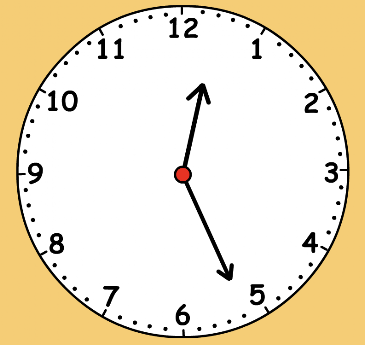ClockWork 12
Set 12 (When shown an analog or digital clock, the student is asked to round the time to the nearest hour or the nearest half hour.)
From Mr. Anker Tests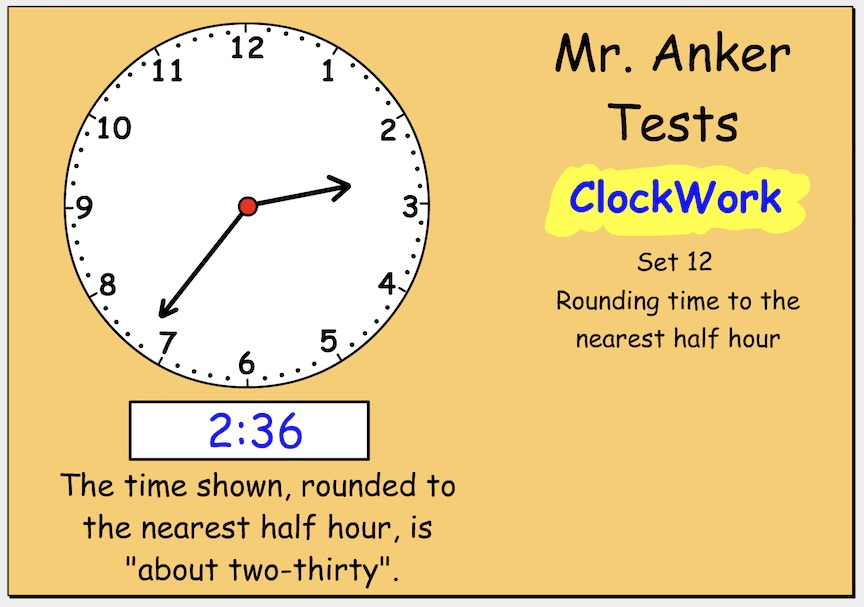1. Round the time shown on the clock below to the nearest half hour.
1 point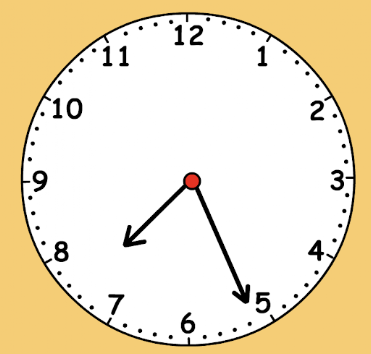2. Round the time shown on the clock below to the nearest half hour. *
1 point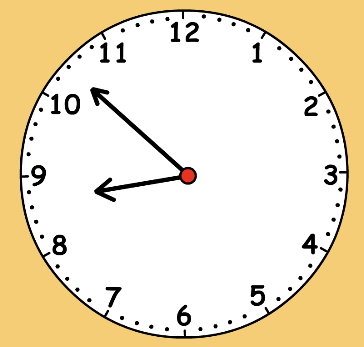3. Round the time shown on the clock below to the nearest half hour. *
1 point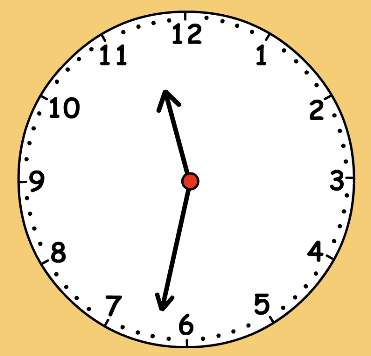4. Round the time shown on the digital clock below to the nearest half hour. *
1 point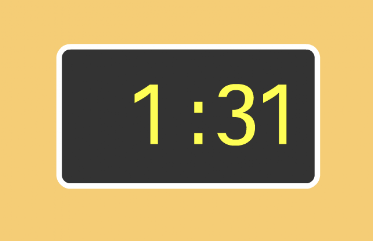5. Round the time shown on the digital clock below to the nearest half hour. *
1 point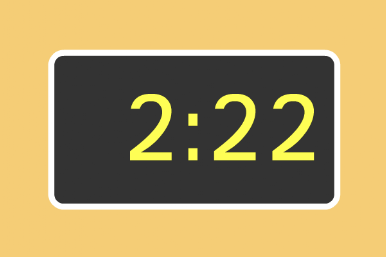6. Round the time shown on the digital clock below to the nearest half hour. *
1 point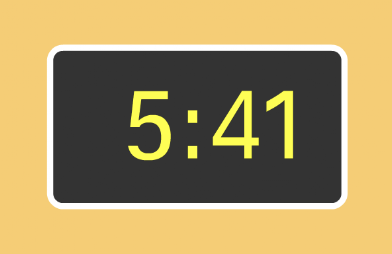7. To the nearest half hour, which of the times listed below properly rounds to 10:30? *
1 point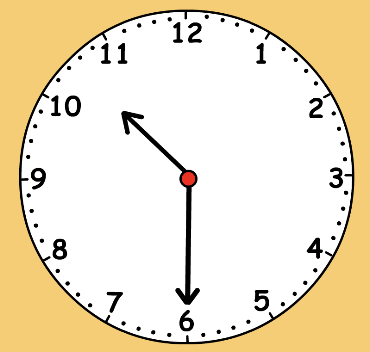8. Which of these times does -not- round to 3:30? *
1 point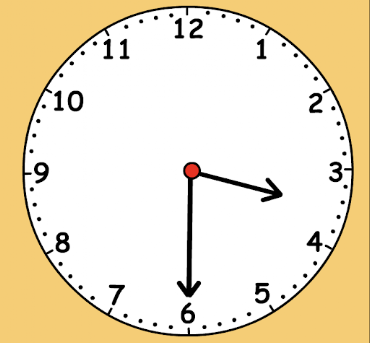9. Round the time displayed on the clock to the nearest half hour. Enter the rounded time on the line below. (Be sure to use a colon to separate the hour and minutes.) *
1 point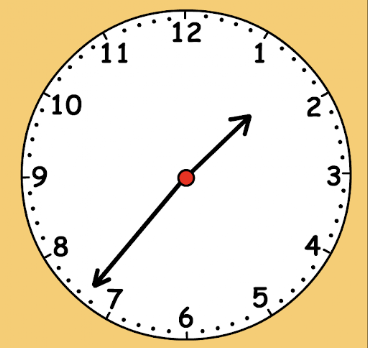10. Round the time displayed on the clock to the nearest half hour. Enter the rounded time on the line below. (Be sure to use a colon to separate the hour and minutes.) *
1 point